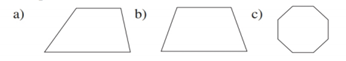Chapter 2.6, Problem 8E### Elementary Geometry for College St...

6th Edition
Daniel C. Alexander + 1 other
ISBN: 9781285195698

#### Solutions

Chapter
Section### Elementary Geometry for College St...

6th Edition
Daniel C. Alexander + 1 other
ISBN: 9781285195698
Textbook Problem
1 views

# Which geometric figures have symmetry with respect to a point?To determine

To choose:

The geometric figures having symmetry with respect to a point.

Explanation

Consider the geometric figure (a).

Figure (1)

Consider arbitrary points A on different point on the polygon.

Figure (2)

Since, there is no point of symmetry exists for the quadrilateral as it is irregular in shape.

The geometric figure (a) is not symmetric about point P.

Consider the geometric figure (b) and consider point P at the centre of the geometric figure with an arbitrary point A

Figure (3)

The geometric figure (b) is not symmetric about point P as the horizontal centre and vertical centre do not coincides for a trapezoid. There is no point of symmetry exists for the trapezoid.

Consider the geometric figure (c) and consider point P at the centre of the geometric figure.

### Still sussing out bartleby?

Check out a sample textbook solution.

See a sample solution

#### The Solution to Your Study Problems

Bartleby provides explanations to thousands of textbook problems written by our experts, many with advanced degrees!

Get Started

#### Differentiate the functions in Problems 3-20. 20.

Mathematical Applications for the Management, Life, and Social Sciences

#### Prove the identity. 47. sec y cos y = tan y sin y

Single Variable Calculus: Early Transcendentals, Volume I

#### is the binomial series for:

Study Guide for Stewart's Single Variable Calculus: Early Transcendentals, 8th

#### In Exercises 110, find the graphical solution to each inequality. 4x80

Finite Mathematics for the Managerial, Life, and Social Sciences

#### The length of a = 4i – j – 2k is: 11 21

Study Guide for Stewart's Multivariable Calculus, 8th